## A 200-turn flat coil of wire 30.0 cm in diameter acts as an antenna for FM radio at a frequency of 100 MHz. The magnetic field of the incomi

Question

A 200-turn flat coil of wire 30.0 cm in diameter acts as an antenna for FM radio at a frequency of 100 MHz. The magnetic field of the incoming electromagnetic wave is perpendicular to the coil and has a maximum strength of 1.00×10−12 T.

(a) What power is incident on the coil?
(b) What average emf is induced in the coil over one-fourth of a cycle?
(c) If the radio receiver has an inductance of 2.50 μH, what capacitance must it have to resonate at 100 MHz?

in progress 0
5 months 2021-08-25T00:08:37+00:00 2 Answers 10 views 0

a) 8.4×10^-12W

b) 5.65 ×10^ -3V

c) 1.01 ×10^ -12 F

Explanation: Given:

Radius r = d/2 = 30/2=15cm= 0.15m

Maximum feild strength = Bo= 10^-12 T

Permiability of vacuum, Uo = 4pi×10^-7

I = CBo/2Uo = (3 ×10^8×(10^-12)^2)/ (4 ×3.142×10^-7)

I = 1.19×10^-10W/m^2

The intensity I = > P/A = p = IA = I(4pi^2)

P = 1.19×10^-10 × 3.142 × (0.15^2)

P = 8.4 × 10^-12W

b) Given: f = 100Mhz= 100×10^6hz

Number of turns in the coil, N = 200

Time of cycle, T = 1/f = 1/ 100×10^6

T = 10^-8 seconds

Change in t= 1/4T = 1/4×10^-8 = 2.5 ×10^-9sec

E = (N×change in B×A)/ change in t

E = (NBopir^2)/change in t

E = (200 ×10^-12× 3.142 ×(0.15^2))/ (2.5×10^-9)

E = 5.65 ×10^-3V

c) Self inductance L = 2.5UH=2.5×10^-6H

Resonant frequency, fo= 1/(2pisqrt(LC))

C= 1/(4×3.142×( 2.5×10^-6)× (100×10^6)^2

C = 1.01×10^-12F

a)P = 8.43 × 10⁻¹² W

b) e =5.65 × 10⁻³ V

c) c = 1.01 × 10⁻¹² F

Explanation:

(a) What power is incident on the coil?

diameter(d) = 30.0 cm = 30 × 10⁻² m

Magnetic strength(B₀) = 1 × 10⁻¹² T

Speed of light(c) = 3 × 10⁸ m/s

Permeability of vacuum(u₀) = 4π × 10⁻⁷ N/A² = 12.57 × 10⁻⁷ N/A²

The magnetic field intensity(I) is given as: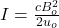The power incident on the coil(P) is given as: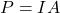Where A is the coil area = πd²/4 = 3.14d²/4

Therefore,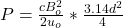substituting values,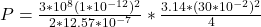P = 8.43 × 10⁻¹² W

(b) What average emf is induced in the coil over one-fourth of a cycle

Frequency (f) = 100 MHz = 100 × 10⁶ Hz

emf(e) = ?

Number of turns(N) = 200 turns

Time(T) = 1/f = 1/(100 × 10⁶) = 10⁻⁸ s

dt =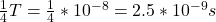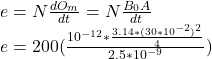e =5.65 × 10⁻³ V

c) If the radio receiver has an inductance of 2.50 μH, what capacitance must it have to resonate at 100 MHz?

Capacitance(c) = ?

Inductance(L) = 2.5 μH = 2.5 × 10⁻⁶ H

resonant frequency(f₀) = 100 MHz = 100 × 10⁶ Hz

At resonant frequency, the capacitance impedance and inductive impedance are equal. Therefore: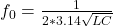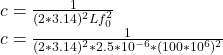c = 1.01 × 10⁻¹² F